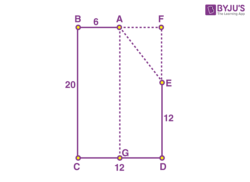# A child walks 6 m towards the west and then 20 in towards south. He then turns east and walks another 12 m. Then he walks 12 m towards the north. How far is he from his initial position?

As per the instructions given, we can draw a diagram as shown belowFrom the given details we note that

AB=6

BC=20

CD=12

DE=12.

From the diagram, we note that

∠ABC=∠BCD=∠CDE=∠ABC=90∘.

Construction: Draw the lines AF and EF which are the extensions of BA and DE respectively such that they meet at point F at the right-angle also.

So, in rectangle FBCD we have four angles equal to 90∘.

We get BC=FD=20,BF=CD=12. (opposite sides are equal in rectangle)

In triangle OED,

EF=FD−DE=20−12=8

AF=BF−AB=12−6=6.
On applying Pythagoras’ theorem For right-angle triangle ΔAEF, we get

AF2+FE2=AE2

=62+82

=36 + 64

AE = 100.

Distance from initial point to terminal point = √100 = 10m(4)(1)